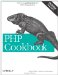# Recipe 4.18. Sorting Multiple Arrays

#### 4.18.1. Problem

You want to sort multiple arrays or an array with multiple dimensions.

#### 4.18.2. Solution

Use array_multisort( ):

To sort multiple arrays simultaneously, pass multiple arrays to array_multisort( ):

`\$colors = array('Red', 'White', 'Blue'); \$cities = array('Boston', 'New York', 'Chicago'); array_multisort(\$colors, \$cities); print_r(\$colors); print_r(\$cities); Array (      => Blue      => Red      => White ) Array (      => Chicago      => Boston      => New York )`

To sort multiple dimensions within a single array, pass the specific array elements:

`\$stuff = array('colors' => array('Red', 'White', 'Blue'),                'cities' => array('Boston', 'New York', 'Chicago')); array_multisort(\$stuff['colors'], \$stuff['cities']); print_r(\$stuff); Array (     [colors] => Array         (              => Blue              => Red              => White         )     [cities] => Array         (              => Chicago              => Boston              => New York         ) )`

To modify the sort type, as in sort( ), pass in SORT_REGULAR, SORT_NUMERIC, or SORT_STRING after the array. To modify the sort order, unlike in sort( ), pass in SORT_ASC or SORT_DESC after the array. You can also pass in both a sort type and a sort order after the array.

#### 4.18.3. Discussion

The array_multisort( ) function can sort several arrays at once or a multidimensional array by one or more dimensions. The arrays are treated as columns of a table to be sorted by rows. The first array is the main one to sort by; all the items in the other arrays are reordered based on the sorted order of the first array. If items in the first array compare as equal, the sort order is determined by the second array, and so on.

The default sorting values are SORT_REGULAR and SORT_ASC, and they're reset after each array, so there's no reason to pass either of these two values, except for clarity:

`\$numbers = array(0, 1, 2, 3); \$letters = array('a', 'b', 'c', 'd'); array_multisort(\$numbers, SORT_NUMERIC, SORT_DESC,                 \$letters, SORT_STRING , SORT_DESC);`

This example reverses the arrays.

Recipe 4.16 for simple sorting and Recipe 4.17 for sorting with a custom function; documentation on array_multisort( ) at http://www.php.net/array-multisort .PHP Cookbook: Solutions and Examples for PHP Programmers
ISBN: 0596101015
EAN: 2147483647
Year: 2006
Pages: 445

Similar book on Amazon